CGAL 4.7 - Triangulated Surface Mesh Simplification
EdgeCollapsableSurfaceMesh Concept Reference

Definition

The concept EdgeCollapsableSurfaceMesh describes the requirements for the type of triangulated surface mesh that can be passed to the simplification algorithm.

The surface mesh must be structurally equivalent to a polyhedral surface having only triangular faces. It can have any number of connected components, boundaries (borders and holes) and handles (arbitrary genus).

Refines:
MutableFaceGraph

Valid Expressions

Let v0v1 an edge of the triangulated surface mesh ecm and v0 and v1 being the source and target vertices of that edge. The surface mesh simplification algorithm requires the call to the function Euler::edge_collapse(e,ecm) to be valid and to return the vertex not removed after collapsing the edge e with the two halfedges v0v1 and v1v0.

For h $$\in \{$$ v0v1,v1v0 $$\}$$, let en and ep be the next and previous edges, that is en = next(h, surface_mesh), ep = prev(h,surface_mesh), and let eno and epo be their opposite edges, that is eno = opposite(en, surface_mesh) and epo = opposite(ep,surface_mesh).

Then, after the collapse of (v0v1,v1v0) the following holds:

• The edge e is no longer in surface_mesh.
• One of $$\{$$v0,v1 $$\}$$ is no longer in surface_mesh while the other remains. Most of the time v0 is the vertex being removed but in some cases removing the edge e requires v1 to be removed. See Figure CollapseFigure5. Let vgone be the removed vertex and vkept be the remaining vertex.
• If e was a border edge, that is get(CGAL::is_border, e, surface_mesh) == true, then next(ep) == en, and prev(en) == ep.
• If e was not a border edge, that is get(is_border, e, surface_mesh) == false, then ep and epo are no longer in surface_mesh while en and eno are kept in surface_mesh.
• For all edges ie in in_edges(vgone,surface_mesh), target(ie,surface_mesh) == vkept and source(opposite(ie,surface_mesh),surface_mesh) == vkept.
• No other incidence information has changed in surface_mesh.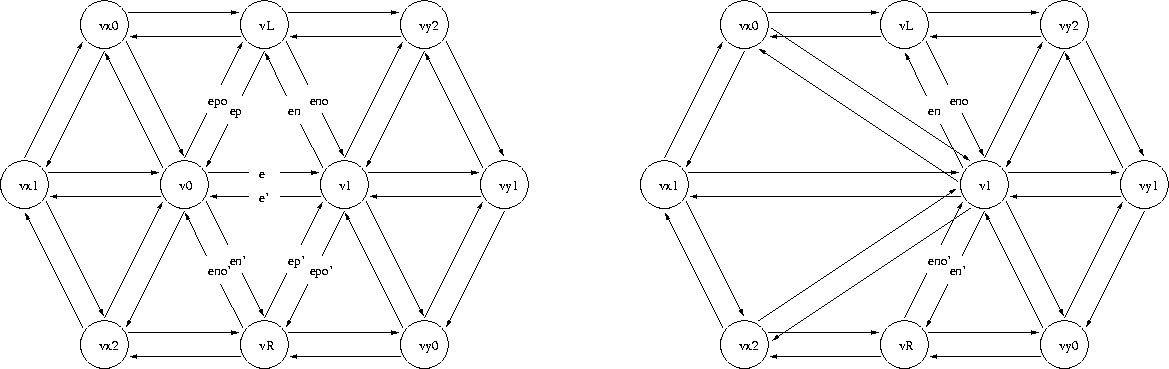General case. The following surface mesh elements are removed: triangles ( $$v0,v1,vL$$) and ( $$v1,v0,vR$$), edges $$(e,e')$$, $$(ep,epo)$$ and $$(ep',epo')$$, and vertex $$v0$$.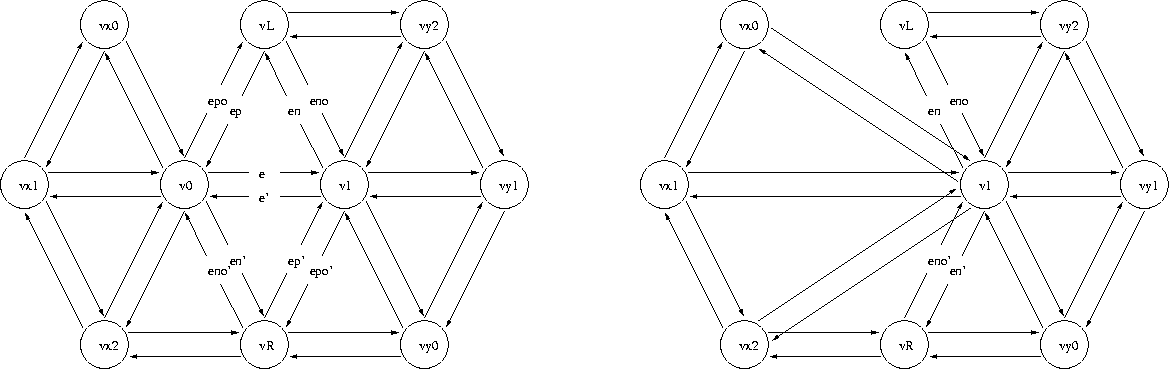When the collapsing edge is not itself a border, but is incident upon a border edge that is removed, the operation is the same as in the general case.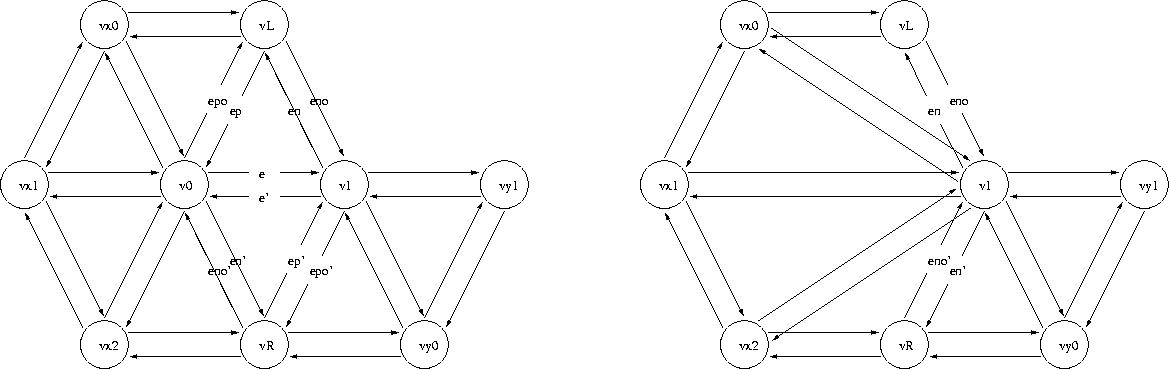When the collapsing edge is not itself a border, but is incident upon a border edge that is not removed, the operation is still the same as in the general case.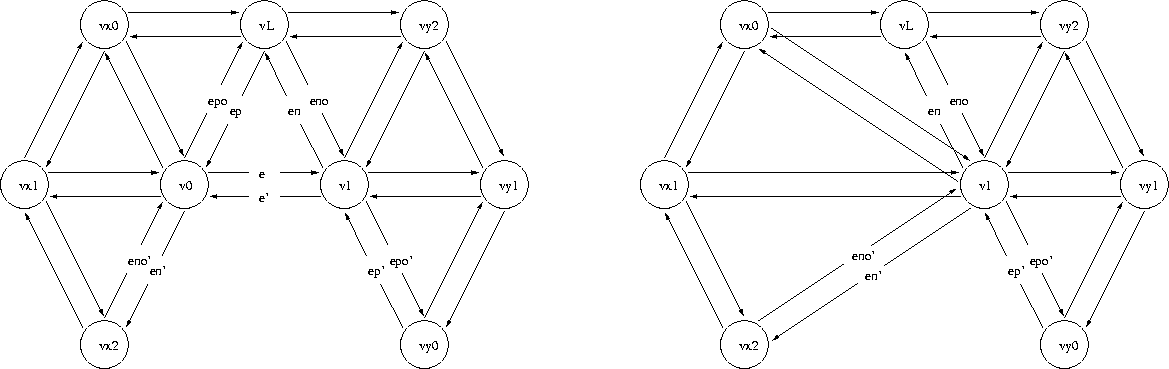When the collapsing edge is itself a border, only 1 triangle is removed. Thus, even if $$(ep',epo')$$ exists, it's not removed.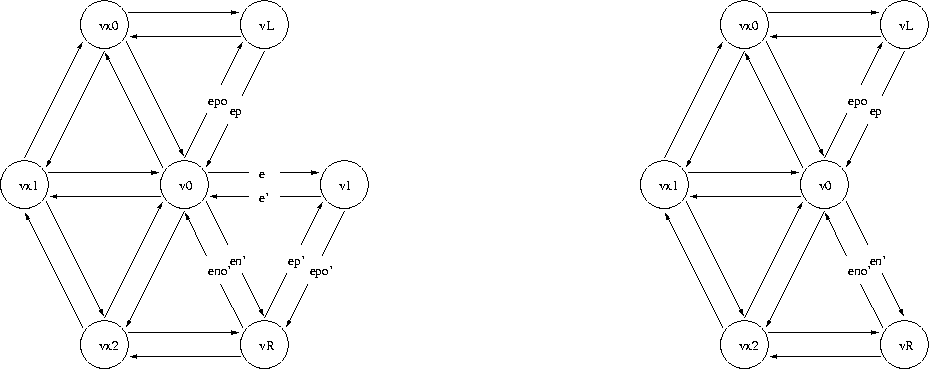This figure illustrates the single exceptional case when removing $$(v0,v1)$$ neccesarily implies removing $$(v1)$$, thus $$(v0)$$ remains.
Has Models:
CGAL::Polyhedron_3<Traits> (If it has only triangular faces), using the specialization boost::graph_traits< CGAL::Polyhedron_3<Traits> > .
boost::graph_traits< CGAL::Polyhedron_3<Traits> >

Related Functions

(Note that these are not member functions.)

template<class EdgeCollapsableSurfaceMesh >
boost::graph_traits
< EdgeCollapsableSurfaceMesh >
::vertex_descriptor
halfedge_collapse (typename boost::graph_traits< EdgeCollapsableSurfaceMesh >::edge_descriptor const &ue, EdgeCollapsableSurfaceMesh &surface_mesh)
Collapses the undirected edge (v0v1,v1v0) replacing it with v0 or v1, as described in the paragraph above. More...

Friends And Related Function Documentation

template<class EdgeCollapsableSurfaceMesh >
 boost::graph_traits< EdgeCollapsableSurfaceMesh >::vertex_descriptor halfedge_collapse ( typename boost::graph_traits< EdgeCollapsableSurfaceMesh >::edge_descriptor const & ue, EdgeCollapsableSurfaceMesh & surface_mesh )
related

Collapses the undirected edge (v0v1,v1v0) replacing it with v0 or v1, as described in the paragraph above.

Precondition
This function requires surface_mesh to be an oriented 2-manifold with or without boundaries. Furthermore, the undirected edge (v0v1,v1v0) must satisfy the link condition , which guarantees that the surface mesh is also 2-manifold after the edge collapse.